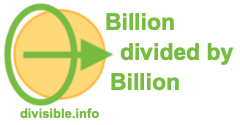Billion divided by Billion CalculatorWelcome to our Billion divided by Billion Calculator. When working with billions, we are dealing with a lot of zeros.

This means that it's easy to make a mistake when you enter your billion numbers into a pocket calculator. In addition, a lot of calculators may not even allow you to enter that many zeros or digits.

Use our Billion divided by Billion Calculator to easily divide billions by billions! Please enter your math problem below so we can solve it for you.

billion divided by  billion

Here are some examples of what our Billion divided by Billion Calculator can calculate for you.

What is 200 billion divided by 7 billion?

What is 44 billion divided by 8 billion?

What is 185 billion divided by 8 billion?

What is 100 billion divided by 7 billion?

What is 120 billion divided by 7 billion?

What is 300 billion divided by 8 billion?

What is 1 billion divided by 10 billion?